# ML | sklearn.linear_model.LinearRegression() in Python

This is Ordinary least squares Linear Regression from sklearn.linear_module.
Syntax :
sklearn.linear_model.LinearRegression(fit_intercept=True, normalize=False, copy_X=True, n_jobs=1):

Parameters :

fit_intercept : [boolean, Default is True] Whether to calculate intercept for the model.
normalize : [boolean, Default is False] Normalisation before regression.
copy_X : [boolean, Default is True] If true, make a copy of X else overwritten.
n_jobs : [int, Default is 1] If -1 all CPU’s are used. This will speedup the working for large datasets to process.

In the given dataset, R&D Spend, Administration Cost and Marketing Spend of 50 Companies are given along with the profit earned. The target is to prepare ML model which can predict the profit value of a company if the value of its R&D Spend, Administration Cost and Marketing Spend are given.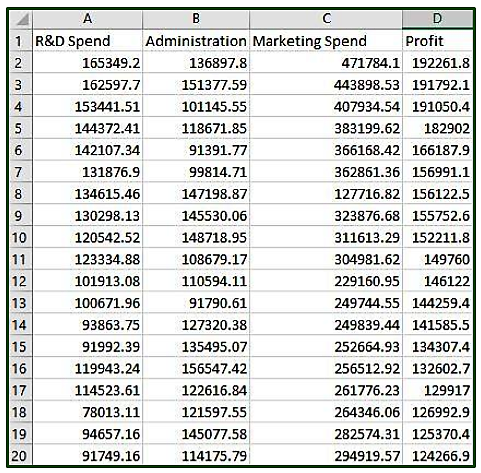Code: Use of Linear Regression to predict the Companies Profit

 `# Importing the libraries ` `import` `numpy as np ` `import` `pandas as pd ` `  `  `# Importing the dataset ` `dataset ``=` `pd.read_csv(``'https://media.geeksforgeeks.org/wp-content/uploads/50_Startups.csv'``) ` `  `  `print` `(``"Dataset.head() \n "``, dataset.head()) ` `  `  `# Input values ` `x ``=` `dataset.iloc[:, :``-``1``].values ` `print``(``"\nFirst 10 Input Values : \n"``, x[``0``:``10``, :]) ` ` `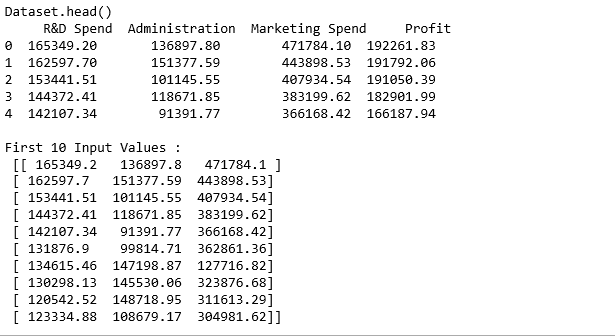`print` `(``"Dataset Info : \n"``) ` `print` `(dataset.info()) `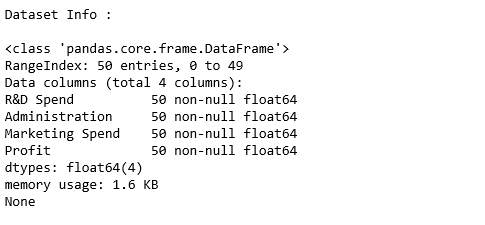`# Input values ` `x ``=` `dataset.iloc[:, :``-``1``].values ` `print``(``"\nFirst 10 Input Values : \n"``, x[``0``:``10``, :]) ` `  `  `  `  `# Output values ` `y ``=` `dataset.iloc[:, ``3``].values  ` `y1 ``=` `y ` `y1 ``=` `y1.reshape(``-``1``, ``1``) ` `print``(``"\n\nFirst 10 Output true value : \n"``, y1[``0``:``10``, :]) `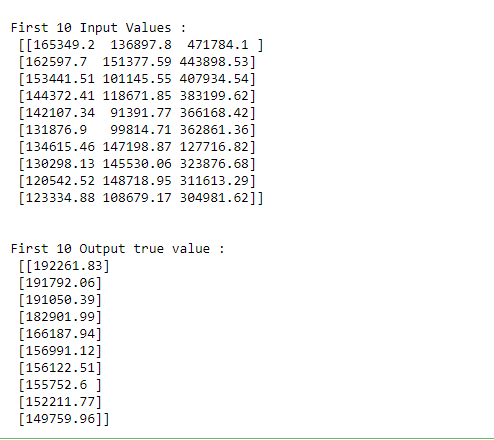`# Dividing input and output data to train and test data ` `# Training : Testing = 80 : 20 ` `from` `sklearn.cross_validation ``import` `train_test_split ` `xtrain, xtest, ytrain, ytest ``=` `train_test_split(x, y, test_size ``=` `0.2``,  ` `                                                     ``random_state ``=` `0``) ` `  `  `# Feature Scaling ` `# Multilinear regression takes care of Feature Scaling ` `# So we need not do it manually  ` `  `  `  `  `# Fitting Multi Linear regression model to training model ` `from` `sklearn.linear_model ``import` `LinearRegression ` `regressor ``=` `LinearRegression() ` `regressor.fit(xtrain, ytrain) ` `  `  `# predicting the test set results ` `y_pred ``=` `regressor.predict(xtest) ` `  `  `y_pred1 ``=` `y_pred ` `y_pred1 ``=` `y_pred1.reshape(``-``1``,``1``) ` `  `  `print``(``"\n   RESULT OF LINEAR REGRESSION PREDICTION : "``) ` `print` `(``"\nFirst 10 Predicted value : \n"``, y_pred1[``0``:``10``, :]) `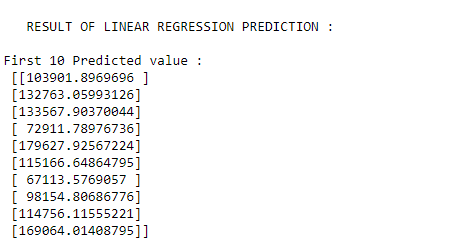Attention geek! Strengthen your foundations with the Python Programming Foundation Course and learn the basics.

To begin with, your interview preparations Enhance your Data Structures concepts with the Python DS Course.

My Personal Notes arrow_drop_upAspire to Inspire before I expire

If you like GeeksforGeeks and would like to contribute, you can also write an article using contribute.geeksforgeeks.org or mail your article to contribute@geeksforgeeks.org. See your article appearing on the GeeksforGeeks main page and help other Geeks.

Please Improve this article if you find anything incorrect by clicking on the "Improve Article" button below.